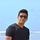cancel
Showing results for
Did you mean:Frequent Visitor

## Product Mix Ratio Calculation

Hi

We have a sales table where we have all the sales of different product types. I can calculate % of total sales. What I want if the product types is 2 series, the % of total should be multiplied by 2, if the product types is 3 series, the % of total should be multiplied by 3, if the product types is 4 series, the % of total should be multiplied by 4. Upon addition of the last column, you get 2.693 which indicates we are selling product types between 2 and 3 (more closer to 3 types)

I am unable to do the final calculation where % of total can be multiplied by product type and then combining all together to get the final number

 Product Types Sales % of Total % multiplied by product type 2 type \$59843 0.46 0.916796887 3 type \$50847 0.39 1.168466771 4 type \$19858 0.15 0.608450532 2.693714189

1 ACCEPTED SOLUTIONSolution Sage

Based on your description, I created data to reproduce your scenario. The pbix file is attached in the end.
https://1drv.ms/u/s!AiUZ0Ws7G26Rh2DVeeDMbnNzhfQo?e=Aq0chX
I created two variants for you

2 REPLIES 2Super User

Hi,

In the Query Editor or via a calculated column formula in the Data model, extract the number from the Product Types column in a seperate column.  Rename this column as Number.  Write this measure

Meausre 1 = sum(Data[Number]))

Measure 2 = SUMX(Values(Data[Product Types]),[% of total]*[Measure 1])

I am assuming that % of total is a measure that you have written.

Regards,
Ashish Mathur
http://www.ashishmathur.comSolution Sage

Based on your description, I created data to reproduce your scenario. The pbix file is attached in the end.
https://1drv.ms/u/s!AiUZ0Ws7G26Rh2DVeeDMbnNzhfQo?e=Aq0chX
I created two variants for you